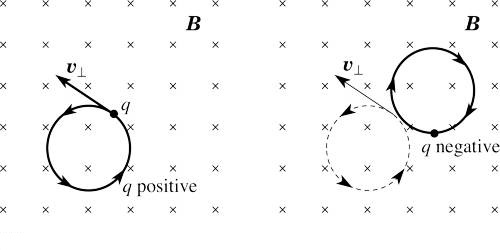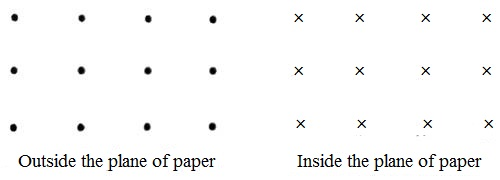# Representation of Direction of Magnetic Field on the Plane of a Paper

Representation of direction of the magnetic field on the plane of a paper:

A paper is a plane, but electromagnetic fields are vectors that exist in all three dimensions. Sometimes they point inwards to the paper and other times they point outwards. In many cases, it is convenient to represent the direction of the magnetic field along the normal to the plane of the paper. There are two directions normal to the paper – one is above the plane and the other is below the plane of the paper. So, whether the magnetic field is outward or inward to the plane of the paper is represented by a common method used everywhere. According to this method dot (.) sign is used to represent the magnetic field outward the plane or towards the reader [Fig. (a)] whereas the cross (x) sign represents the inward direction of the field [Fig. (b)]. One can immediately identify the direction of the magnetic field by looking at these signs. In terms of the direction, the cross(x) means out of the plane i.e. when an object is moving out of the plane and dot(.) means entering into a plane. This is the answer if u mean in terms of CURRENT’s direction.Work: A charge is located in a magnetic field. Will the magnetic field exerts any force on \ the charge when (i) charge is stationary, (ii) when a charge is in motion, and along the direction of the magnetic field (iii) when the charge is in the motion perpendicular to the magnetic field?

(1) The magnetic field will not exert any force on a stationary charge.

(ii) The magnetic field exerts the force on a moving charge. The magnetic field also is generated. But since the charge is in motion along the direction of the magnetic field, so no force will be exerted.

(iii) When the charge is in the motion perpendicular to the direction of the magnetic field, then force on the charge is = Bev, here B = magnetic field, e = charge, and v = velocity of the charge.

The properties of magnetic field lines can be summarized by these rules:

• The direction of the magnetic field is tangent to the field line at any point in space. A small compass will point in the direction of the field line.
• The strength of the field is proportional to the closeness of the lines. It is exactly proportional to the number of lines per unit area perpendicular to the lines (called the areal density).
• Magnetic field lines can never cross, meaning that the field is unique at any point in space.
• Magnetic field lines are continuous, forming closed loops without beginning or end. They go from the north pole to the south pole.